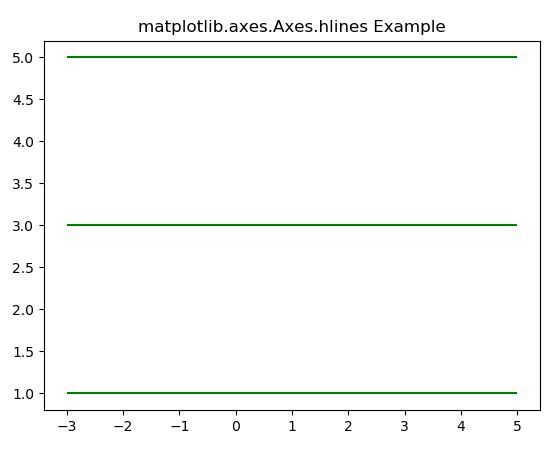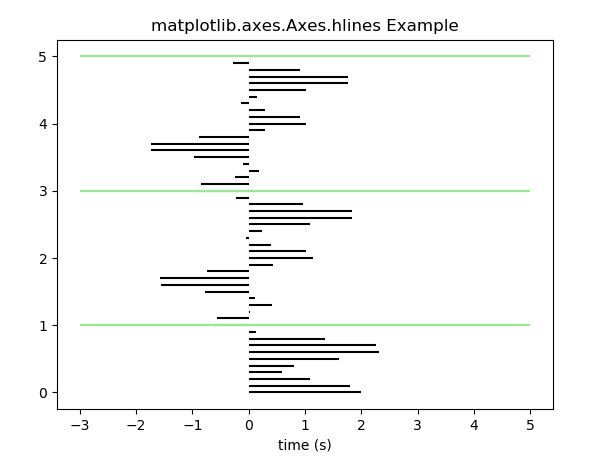Skip to content
Related Articles
Matplotlib.axes.Axes.hlines() in Python
• Last Updated : 13 Apr, 2020

Matplotlib is a library in Python and it is numerical – mathematical extension for NumPy library. The Axes Class contains most of the figure elements: Axis, Tick, Line2D, Text, Polygon, etc., and sets the coordinate system. And the instances of Axes supports callbacks through a callbacks attribute.

## matplotlib.axes.Axes.hlines() Function

The Axes.hlines() function in axes module of matplotlib library is used to Plot vertical lines at each y from xmin to xmax.

Syntax: Axes.hlines(self, y, xmin, xmax, colors=’k’, linestyles=’solid’, label=”, *, data=None, **kwargs)

Parameters: This method accept the following parameters that are described below:

• y: This parameter is the sequence of y-indexes where to plot the lines.
• xmin, xmax: These parameter contains an array.And they represents the beginning and end of each line.
• colors: This parameter is an optional parameter. And it is the color of the lines with default value k.
• linetsyle: This parameter is also an optional parameter. And it is used to represent the linestyle{‘solid’, ‘dashed’, ‘dashdot’, ‘dotted’}.
• label: This parameter is also an optional parameter.It is the label of the plot.

Returns: This returns the LineCollection.

Below examples illustrate the matplotlib.axes.Axes.hlines() function in matplotlib.axes:

Example #1:

 `# Implementation of matplotlib function``      ` `import` `numpy as np``from` `matplotlib ``import` `patches``import` `matplotlib.pyplot as plt``  ` `fig, ax ``=` `plt.subplots()``ax.hlines([``1``, ``3``, ``5``], ``-``3``, ``5``, color ``=``"green"``)``ax.set_title(``'matplotlib.axes.Axes.hlines Example'``)`` ` `plt.show()`

Output:Example #2:

 `# Implementation of matplotlib function``      ` `import` `numpy as np``from` `matplotlib ``import` `patches``import` `matplotlib.pyplot as plt``  ` `t ``=` `np.arange(``0.0``, ``5.0``, ``0.1``)``s ``=` `np.exp(``-``t) ``+` `np.cos(``3` `*` `np.pi ``*` `t) ``+` `np.sin(np.pi ``*` `t)``nse ``=` `np.random.normal(``0.0``, ``0.8``, t.shape) ``*` `s`` ` `fig, ax ``=` `plt.subplots()`` ` `ax.hlines(t, [``0``], s)``ax.set_xlabel(``'time (s)'``)``ax.hlines([``1``, ``3``, ``5``], ``-``3``, ``5``, color ``=``"lightgreen"``)``ax.set_title(``'matplotlib.axes.Axes.hlines Example'``)`` ` `plt.show()`

Output:Attention geek! Strengthen your foundations with the Python Programming Foundation Course and learn the basics.

To begin with, your interview preparations Enhance your Data Structures concepts with the Python DS Course. And to begin with your Machine Learning Journey, join the Machine Learning – Basic Level Course

My Personal Notes arrow_drop_up